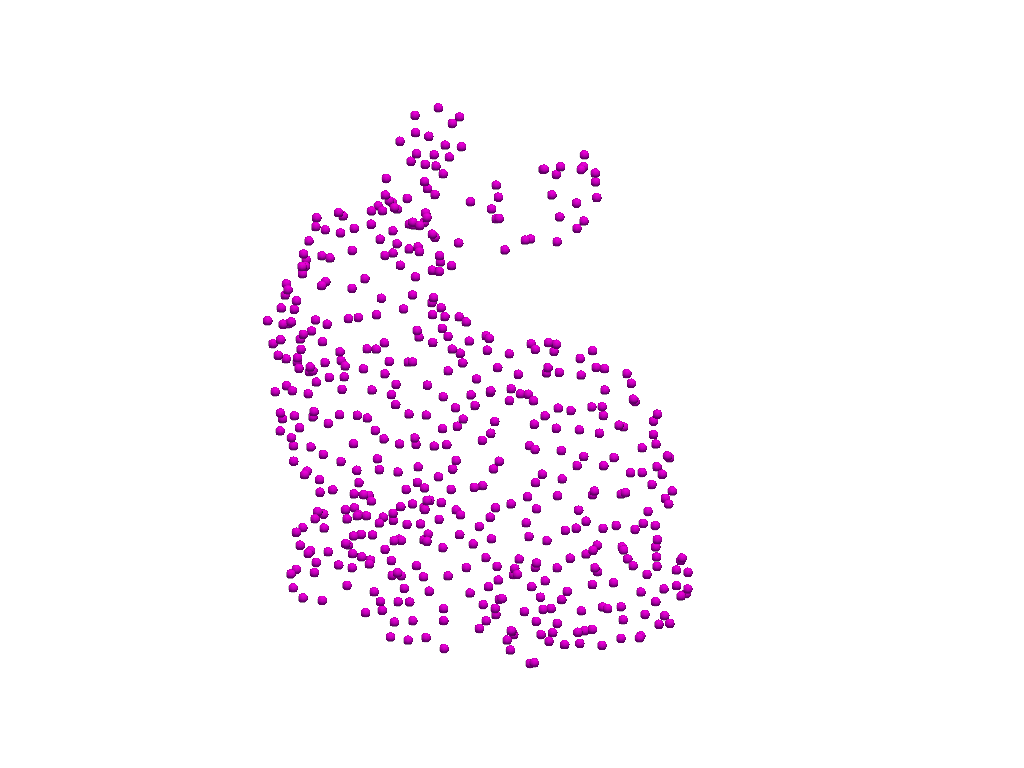# Visible Vertices#

Display vertices on a mesh in the same fashion as edge visibility.

```import pyvista as pv
from pyvista import examples

cpos = [(0.036, 0.367, 0.884), (0.024, 0.033, -0.022), (-0.303, 0.895, -0.325)]
```

We can easily display all vertices of a mesh with a `points` style representation when plotting:

```pl = pv.Plotter()
pl.show(cpos=cpos)
```However, we often want to see the vertices of a mesh rendered atop the surface geometry. Much like how we can render the edges of a mesh:

```pl = pv.Plotter()
pl.show(cpos=cpos)
```In order to display the vertices atop a mesh’s surface geometry, simply pass `show_vertices=True` to render them along side the original geometry.

Note

Vertex styling can be changed using `vertex_color`, `vertex_opacity`, and `vertex_style`.

```pl = pv.Plotter()
mesh,
show_edges=True,
vertex_color='magenta',
render_points_as_spheres=True,
point_size=10,
show_vertices=True,
)
pl.show(cpos=cpos)
```If you wish to have further control over the way surface points are plotted alongside the surface geometry, extract the surface points and plot them separately.

The first step is to extract the outer surface geometry of the mesh then grab all the points of that extraction.

```surf_points = mesh.extract_surface().points
```

Now that we have the vertices extracted, we can use `add_points()` to render them along side the original geometry.

Color the points by their Y position.

```pl = pv.Plotter()
surf_points,
color='magenta',
render_points_as_spheres=True,
point_size=10,
scalars=surf_points[:, 1],
lighting=False,
show_scalar_bar=False,
)
pl.show(cpos=cpos)
```Total running time of the script: ( 0 minutes 0.805 seconds)

Gallery generated by Sphinx-Gallery